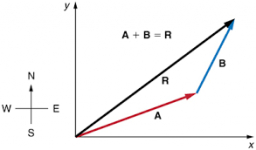# Components 67664

The force R = 12 N is divided into two components, F1 and F2. Their directions make angles α = 30 °, β = 45 ° with the direction R. What are the components F1, F2?

F1 =  6.2117 N
F2 =  8.7846 N

### Step-by-step explanation:Did you find an error or inaccuracy? Feel free to write us. Thank you!

Tips for related online calculators
Our vector sum calculator can add two vectors given by their magnitudes and by included angle.

#### Grade of the word problem:

We encourage you to watch this tutorial video on this math problem: